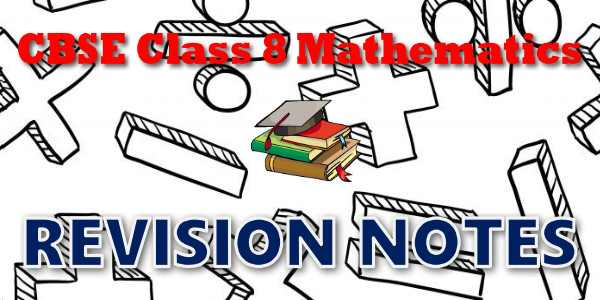No products in the cart.

# CBSE - Class 08 - Mathematics - CBSE Revision Notes## CBSE, JEE, NEET, CUET

Question Bank, Mock Tests, Exam Papers

NCERT Solutions, Sample Papers, Notes, Videos

## CBSE Revision Notes for Class 08 Mathematics

Rational Numbers
Rational Numbers## myCBSEguide App

Complete Guide for CBSE Students

NCERT Solutions, NCERT Exemplars, Revison Notes, Free Videos, CBSE Papers, MCQ Tests & more.

Linear Equations in One Variable
Linear Equations In One Variables
Practical Geometry (Deleted)
Practical Geometry
Data Handling
Data Handling
Squares and Square Roots
Squares And Square Roots
Cubes and Cube Roots
Cubes And Cube Root
Comparing Quantities
Comparing Quantities
Algebraic Expressions and Identities
Algebraic Expressions And Identities
Visualising Solid Shapes (Deleted)
Visualising Solid Shapes
Mensuration
Mensuration
Exponents and Powers
Exponents And Powers
Direct and Inverse Proportions
Direct And Inverse Proportions
Factorisation
Factorisation
Introduction to Graphs
Introduction To Graphs
Playing with Numbers (Deleted)
Playing With Numbers### Student Subscription

##### Unlock the exclusive content designed for the toppers## myCBSEguide App

Complete Guide for CBSE Students

NCERT Solutions, NCERT Exemplars, Revison Notes, Free Videos, CBSE Papers, MCQ Tests & more.

# CBSE Revision Notes for class 8 Mathematics## CBSE Class 8 Notes and Key Points

• CBSE Revision notes for Class 8 Mathematics PDF
• CBSE Revision notes Class 8 Mathematics – CBSE
• CBSE Revisions notes and Key Points Class 8 Mathematics
• Summary of the NCERT books all chapters in Mathematics class 8
• Short notes for CBSE class 8th Mathematics
• Key notes and chapter summary of Mathematics class 8
• Quick revision notes for CBSE exams

## CBSE Class 8 Mathematics Chapter-wise Revision Notes

• Chapter 1 Rational Numbers
• Chapter 2 Linear Equations in One Variable
• Chapter 4 Practical Geometry
• Chapter 5 Data Handling
• Chapter 6 Squares and Square Roots
• Chapter 7 Cubes and Cube Roots
• Chapter 8 Comparing Quantities
• Chapter 9 Algebraic Expressions and Identities
• Chapter 10 Visualising Solid Shapes
• Chapter 11 Mensuration
• Chapter 12 Exponents and Powers
• Chapter 13 Direct and Inverse Proportions
• Chapter 14 Factorisation
• Chapter 15 Introduction to Graphs
• Chapter 16 Playing with Numbers

Key Notes for CBSE Board Students for Class 08 Important topics of all subjects are given in these CBSE notes. These notes will provide you overview of the chapter and important points to remember. These are very useful summary notes with neatly explained examples for best revision of the book.

## CBSE Class-08 Revision Notes and Key Points

CBSE class-08  Key points and summary of the lessons is given under this section for Science, Mathematics, Hindi, English, Social Science and other subjects. The notes includes all concepts given in NCERT books and syllabus issued by CBSE for class-08. Key notes are 'to the point' capsules for quick revision of the chapter. We have covered the whole syllabus in these notes.

CBSE Class 8 Mathematics
Revision Notes
Chapter – 01
Rational Numbers

• Rational numbers are closed under the operations of addition, subtraction and multiplication.
• The operations addition and multiplication are
(i) commutative for rational numbers.
(ii) associative for rational numbers.
• The rational number 0 is the additive identity for rational numbers.
• The rational number 1 is the multiplicative identity for rational numbers.
• The additive inverse of the rational number abab is abab and vice-versa.
• The reciprocal or multiplicative inverse of the rational number abab is cdcd if ababxcdcd=1
• Distributivity   of rational numbers: For all rational numbers a, b and c,
a(b + c) = ab + ac    and  a(b – c) = ab – ac
• Rational numbers can be represented on a number line.
• Between any two given rational numbers there are countless rational numbers. The idea of mean helps us to find rational numbers between two rational numbers.
• Positive Rationals: Numerator and Denominator both are either positive or negative. Example: 47,−3−447,−3−4
• Negative Rationals: Numerator and Denominator both are of opposite signs. Example: −211,4−9−211,4−9
• Mulitiplicative Inverse (reciprocal): ababxcdcd=1=cdcdxabab where cdcd is the reciprocal of abab. Zero has no reciprocal. The reciprocal of 1 is1 and of -1 is -1.## myCBSEguide

Trusted by 1 Crore+ Students## Test Generator

Create papers online. It's FREE.## CUET Mock Tests

75,000+ questions to practice only on myCBSEguide app